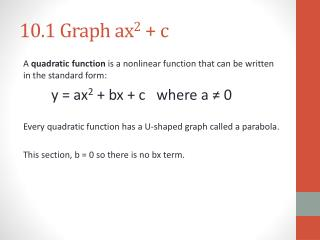DownloadDownload Presentation10.1 Graph ax 2 + c

# 10.1 Graph ax 2 + c

Download Presentation## 10.1 Graph ax 2 + c

- - - - - - - - - - - - - - - - - - - - - - - - - - - E N D - - - - - - - - - - - - - - - - - - - - - - - - - - -
##### Presentation Transcript

1. 10.1 Graph ax2 + c A quadratic function is a nonlinear function that can be written in the standard form: y = ax2 + bx + c where a ≠ 0 Every quadratic function has a U-shaped graph called a parabola. This section, b = 0 so there is no bx term.

2. Given: y = 2x2 a. Complete the table of values b. Identify its domain and range c. Compare to the parent function graph of y = x2 d. Graph the function and the parent function a. D: R: c. The function is:

3. Given: y = - 1/4 x2 a. Complete the table of values b. Identify its domain and range c. Compare to the parent function graph of y = x2 d. Graph the function and the parent function a. D: R: c. The function is:

4. Given: y = x2 - 2 a. Complete the table of values b. Identify its domain and range c. Compare to the parent function graph of y = x2 d. Graph the function and the parent function a. D: R: c. The function is:

5. Given: y = -2x2 + 4 a. Complete the table of values b. Identify its domain and range c. Compare to the parent function graph of y = x2 d. Graph the function and the parent function a. D: R: c. The function is:

6. Example 4b.- MORE PRACTICE Given: y = 1/3x2 - 3 a. Complete the table of values b. Identify its domain and range c. Compare to the parent function graph of y = x2 d. Graph the function and the parent function a. D: R: c. The function is:

7. Example 6- Extended Response Egg Contest For an engineering contest, you have to create a container for an egg so that the container can be dropped from a height of 30 feet without breaking the egg. The height y (in feet) of the dropped container is given by the function y = -16t2 + 30 where t is the time (in seconds since the container is dropped. a. Graph the function. b. Identify the range of the function in this situation. c. Find the egg’s height at 1 second. d. Use the graph to estimate when the egg is at a height of 10 feet. e. Use the graph to estimate when the egg is on the ground.

8. Example 6 Table/Graph R: Height at 1 sec. Approximate time when height is 10 feet. Approximate time when height is 0 feet (ground). 5 4 3 2 1